• 模式识别与智能计算》基于PCA的模板匹配法 《模式识别与智能计算》基于PCA的模板匹配法 基于PCA的模板匹配法 1.实现步骤 2.编程代码 3.运行方法和结果 前言：此篇博客主要介绍《模式识别与智能计算...
《模式识别与智能计算》基于PCA的模板匹配法

《模式识别与智能计算》基于PCA的模板匹配法
基于PCA的模板匹配法
1.实现步骤
2.编程代码
3.运行方法和结果

前言：此篇博客主要介绍《模式识别与智能计算》中代码如何使用。

先决条件：《模式识别与智能计算》—MATLAB技术实现 软件配置
《模式识别与智能计算》第2章 特征的选择与优化

我假设你环境配置出错！不能运行手写识别程序。但是你要下载成功此数据：下载：《模式识别与智能计算》MATLAB技术实现（光盘文件）之后，可以在 手写数字分类文件夹内找到templet.mat数据。
软件选择0类别对应templet中的pattern(1).feature；

需要条件：1. templet.mat
2.allsamples.mat：此文件下载后，内容是我自己的手写体识别；
2中数据可自行保存，保存方法如下：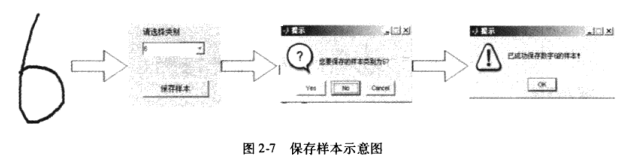allsamples.mat解释：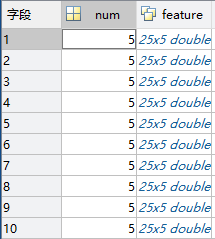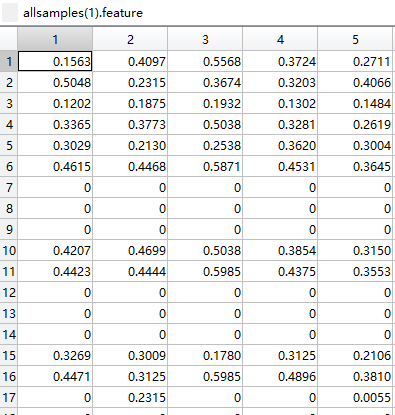左图，分别是0~9结构体，每个数字有5个样本；右图，打开数字0样本，25*5，每一列为1个样本；

基于PCA的模板匹配法

在使用模板匹配法之前，先对特征进行主成分分析。按照一定的贡献值，提取前m个主分量，用较低维数的特征来进行分类。

1.实现步骤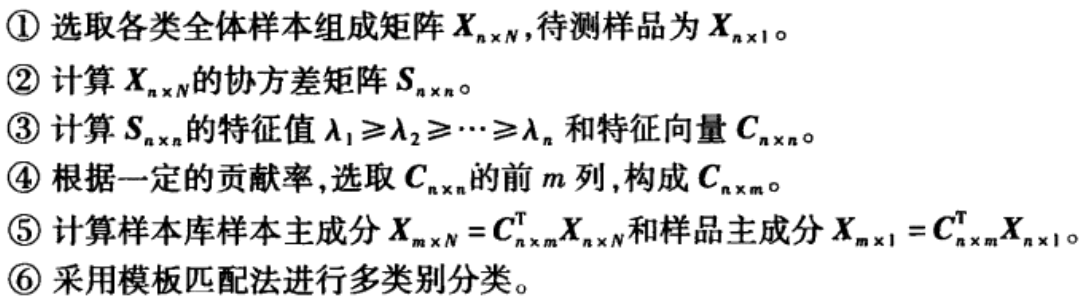2.编程代码

pcapro.m文件：

%  %  %  %  %  %  %  %  %  %  %  %  %  %  %  %
%函数名称：：pcapro()
%参数：sample：待识别样品特征
%返回值：y1:样品库经过主成分分析后的主分量矩阵；y2：样品库经过主成分分析后的主分量向量
%函数功能：对样品库和待测样品用主成分分析法进行降维
%  %  %  %  %  %  %  %  %  %  %  %  %  %  %  %
function [y1,y2]=pcapro(sample)
mixedsig=[];
sum1=0;
%将所有类别的所有样品合并到mixedsig
for i=1:10
sum1=sum1+pattern(i).num;
mixedsig=[mixedsig pattern(i).feature];
end
[Dim,NumofSampl]=size(mixedsig);%Dim为特征数，NumofSampl为样品总个数
dsig_cov=cov(mixedsig');%求mixedsig的协方差矩阵
%利用pcacov()函数从大到小排好序的协方差矩阵的特征值latent和相应特征向量pc
[pc,latent,tspuare]=pcacov(dsig_cov);
temp=0;con=0;m=0;
%根据贡献率取舍特征向量
sum2=sum(latent);
for i=1:25
if(con<0.9)
temp=temp+latent(i);
con=temp/sum2;
m=m+1;
else
break;
end
end
pc(:,m+1:25)=[];
%求待测样品主成分
x=sample'*pc;       %此处和书中不同，因为我的数据是按照列 如按行可改为x=sample*pc;
%求样品库品成分
y=mixedsig'*pc;
y1=y';
y2=x';


pcaneartemplet.m文件：

%函数名称：pcaneartemplet()
%参数：sample：待识别样品特征
%返回值：y:待识别样品所属类别
%函数功能：按照使用PCA的模板匹配法计算待测样品与样品库中的样品相似度
%  %  %  %  %  %  %  %  %  %  %  %  %  %  %  %  %
function y=pcaneartemplet(sample);
clc;
%对样品和样品库进行主成分分析
[pcapat,pcasamp]=pcapro(sample);
temp=0;
for i=1:10
pattern(i).feature=pcapat(:,temp+1:temp+pattern(i).num);
temp=temp+pattern(i).num;
end
d=0;%距离
min=[inf,0];
for i=1:10
for j=1:pattern(i).num
%计算待测样品与样品库间的最小距离
d=sqrt(sum((pattern(i).feature(:,j)-pcasamp).^2));
%求最小距离及其类号
if min(1)>d
min(1)=d;
min(2)=i-1;
end
end
end
%输出类别
y=min(2);

3.运行方法和结果

将pcaneartemplet.m、pcapro.m、templet.mat和allsamples.mat放同一目录下。输入：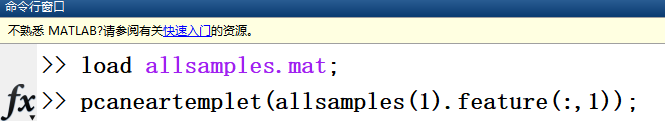运行结果：

ans = 0;

因为输入的样本为0，分类结果也为0，故分类正确！！！
展开全文PCA
• 重点是在软件中的实现，matlab. 《模式识别与智能计算》 杨淑莹 2008.1 电子工业出版社

重点是在软件中的实现，matlab.

《模式识别与智能计算》
杨淑莹
2008.1
电子工业出版社


展开全文• 模式识别与智能计算》模板匹配法 《模式识别与智能计算》模板匹配法 理论基础 两类别 实现步骤 编程代码 运行结果 模板匹配法应该是最简单的模式识别方法。可谁让我是小白呢，学习一下。 理论...
《模式识别与智能计算》模板匹配法

《模式识别与智能计算》模板匹配法
理论基础
两类别

实现步骤
编程代码
运行结果

模板匹配法应该是最简单的模式识别方法。可谁让我是小白呢，学习一下。

理论基础

​   就是把未知样品和一个标准模板相比，看它们是否相同或相似。主要讨论两类别情况。

两类别

​   设有两个标准样品模板为A$A$$A$和B$B$$B$，其特征向量为n$n$$n$维特征：XA=(xA1,xA2,…,xAn)T${X}_{A}=\left({x}_{A1},{x}_{A2},\dots ,{x}_{An}{\right)}^{T}$$X_A=(x_{A1},x_{A2},\dots,x_{An})^T$和XB=(xB1,xB2,…,xBn)T${X}_{B}=\left({x}_{B1},{x}_{B2},\dots ,{x}_{Bn}{\right)}^{T}$$X_B=(x_{B1},x_{B2},\dots,x_{Bn})^T$。任何一个待识别的样品X，其特征向量为：X=(x1,x2,…,xn)T$X=\left({x}_{1},{x}_{2},\dots ,{x}_{n}{\right)}^{T}$$X=(x_1,x_2,\dots,x_n)^T$，那么，他是A$A$$A$还是B$B$$B$ ?

​   用模板匹配方法来识别，若X=XA$X={X}_{A}$$X=X_A$，则该样品为A$A$$A$，若X=XB$X={X}_{B}$$X=X_B$，则该样品为B$B$$B$。最简单的识别方法就是利用距离来判别。

最小距离判别法：如果X距离XA${X}_{A}$$X_A$比距离XB${X}_{B}$$X_B$近，则X$X$$X$属于XA${X}_{A}$$X_A$，否则属于XB${X}_{B}$$X_B$。

任意两点X,Y$X,Y$$X,Y$之间的距离：
d(X,Y)=[∑i=1n(xi−yi)2]12$d\left(X,Y\right)=\left[\sum _{i=1}^{n}\left({x}_{i}-{y}_{i}{\right)}^{2}{\right]}^{\frac{1}{2}}$
d(X,Y)=[\sum_{i=1}^{n}(x_i-y_i)^2]^{\frac{1}{2}}

根据距离远近可作为判据，构成距离分类器，其判别法则为：
{d(X,XA)<d(X,XB)⇒X∈Ad(X,XA)>d(X,XB)⇒X∈B$\left\{\begin{array}{l}d\left(X,{X}_{A}\right)d\left(X,{X}_{B}\right)⇒X\in B\end{array}$
\begin{cases}
d(X,X_A)<d(X,X_B)\Rightarrow X \in A \\
d(X,X_A)>d(X,X_B)\Rightarrow X \in B \\
\end{cases}

实现步骤

待测样品X$X$$X$与训练集里每个样品Xi${X}_{i}$$X_i$的距离为d(X,Y)=[∑ni=1(xi−yi)2]12$d\left(X,Y\right)=\left[\sum _{i=1}^{n}\left({x}_{i}-{y}_{i}{\right)}^{2}{\right]}^{\frac{1}{2}}$$d(X,Y)=[\sum_{i=1}^{n}(x_i-y_i)^2]^{\frac{1}{2}}$;
循环计算待测样品和训练集中各已知样品之间的距离，找出距离待测样品最近的已知样品，该已知样品的类别就是待测样品的类别。

编程代码

%函数名称：neartemplet()
%参数：sample:待识别样品特征
%返回值：y:待识别样品所属类别
%函数功能：按照模板匹配法计算待测样品与样品库中的样品相似度
function y=neartemplet(sample);
clc;
d=0;%距离
min=[inf,0];
for i=1:10
for j=1:pattern(i).num
%计算待测样品与样品库样本间的最小距离
d=sqrt(sum((pattern(i).feature(:,j)-sample').^2));
%求最小距离及其类号
if min(1)>d
min(1)=d;
min(2)=i-1;
end
end
end
%输出类别
y=min(2);

其中：d(X,Y)=[∑ni=1(xi−yi)2]12$d\left(X,Y\right)=\left[\sum _{i=1}^{n}\left({x}_{i}-{y}_{i}{\right)}^{2}{\right]}^{\frac{1}{2}}$$d(X,Y)=[\sum_{i=1}^{n}(x_i-y_i)^2]^{\frac{1}{2}}$代码如下：

d=sqrt(sum((pattern(i).feature(:,j)-sample').^2));

运行结果

sample来源：手写识别题个人样本
templet来源：《模式识别与智能计算》MATLAB技术实现（光盘文件）
不做过多解释了，详情请看此系列博客。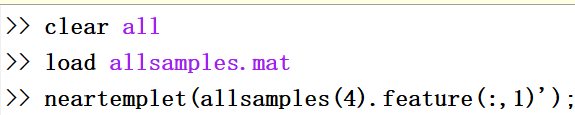ans = 3

因为使用数字3的样本，故结果正确。
展开全文模板匹配法 MATLAB
• 模式识别与智能计算》—MATLAB技术实现 《模式识别与智能计算》—MATLAB技术实现 配置资源： 光盘内容： 软件安装说明： 打开MATLAB 2007： 例程： 前言：前几天在网上买了第3版，翻来一看发现代码和...
《模式识别与智能计算》—MATLAB技术实现

《模式识别与智能计算》—MATLAB技术实现
配置资源：
光盘内容：
软件安装说明：
打开MATLAB 2007：
例程：

前言：前几天在网上买了第3版，翻来一看发现代码和环境都无法配置，给技术人员发邮件也没人回复。想运行书中的代码也无从下手，在网上找了几天资源终于测试成功。

配置资源：

由于第2版和第3版没有提供软件配置说明，只有第1版提供配置软件。

1.Matlab R2007b：链接: https://pan.baidu.com/s/10MTzEe9aWF5ciumIm1g3fw 密码: hodz
2. 《模式识别与智能计算》MATLAB技术实现（光盘文件）

注：本人win7环境

为什么要安装2007版本？高版本MATLAB运行会出错，如下：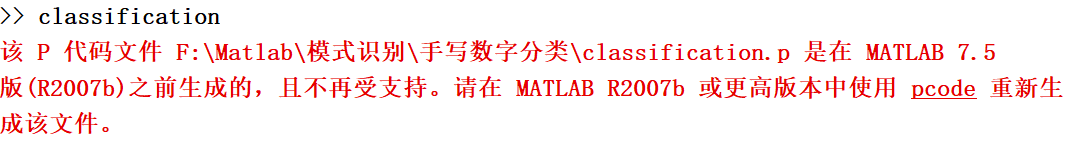第3版书中代码：《模式识别与智能计算》MATLAB技术实现(第2版).杨淑莹

光盘内容：

光盘内容：
本光盘主要由两部分内容组成：
一、手写数字分类软件。
二、聚类分析软件。

软件安装说明：

一、手写数字分类软件。
1)  打开“手写数字分类软件”文件夹，点击“手写数字分类.msi”安装程序，在安装向导中选择安装目录，安装完成。
2)  打开Matlab软件程序，将当前工作目录设为“手写数字分类”的安装目录，在命令行中输入“classification”命令，即可打开手写数字分类软件。
二、聚类分析软件。
1)  打开“聚类分析软件”文件夹，点击“聚类分析.msi”安装程序，在安装向导中选择安装目录，安装完成。
2)  打开Matlab软件程序，将当前工作目录设为“聚类分析”的安装目录，在命令行中输入“Cluster”命令，即可打开聚类分析软件。

打开MATLAB 2007：

设置路径：File→Set Path→将上述程序安装目录载入。
MATLAB命令行窗口输入:classification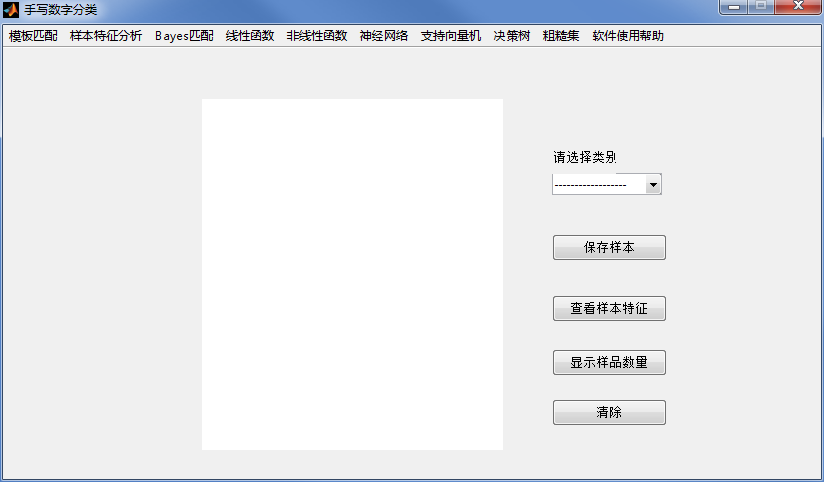例程：

鼠标左键写入：0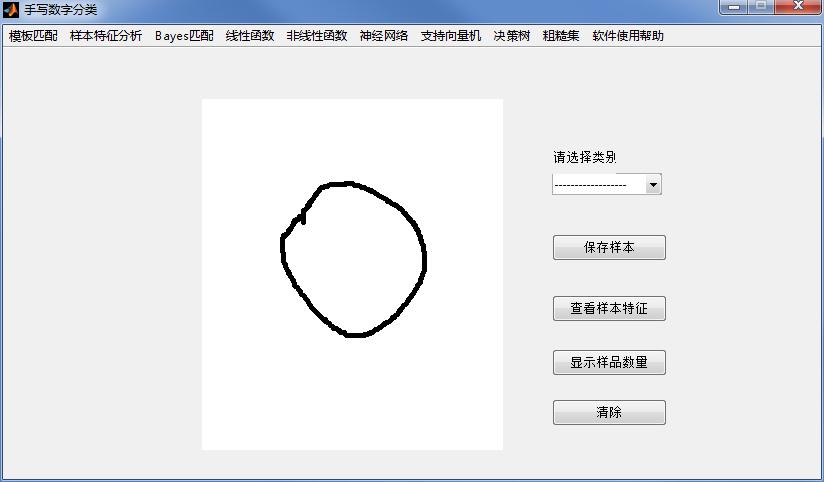点击：模板匹配→最近邻模板匹配法展开全文• 模式识别与智能计算——matlab技术实现（第2版）》广泛吸取统计学、神经网络、数据挖掘、机器学习、人工智能、群智能计算等学科的先进思想和理论，将其应用到模式识别领域中；以一种新的体...数据挖掘 大数据 人工智能
• 最近开始专心学习机器学习、模式识别算法，主要通过斯坦福大学Andrew Ng老师的《机器学习》课程和《模式识别与智能计算-matlab技术实现》这本书进行学习。以模式识别为主线进行学习。那本书可在此下载 ...
• 因为突然要用到特征提取、模式识别的东西，所以现在开始补习这方面的理论知识，因为之前没怎么去看模式识别，下载了书上的源代码错误率太高了，，，应该是这个写代码的人的书写习惯和我不一样 所以我想着 我也写十...
• 1.1模式识别的基本概念  1.模式的描述方法  一个待分类的对象就是一个样本。  必须确定一些识别有关的因素作为分类研究的根据，每一个因素成为一个特征；模式就是样品所具有特征的描述。  模式中抽取的多个...
•  模式识别算法的设计都是强调“最优”或“最佳”，希望所设计的系统在性能上最优。这种“最优”是针对某一种设计原则而言的，及准则。 1.3.1分类器设计准则如下：  1）最小误差率准则；  2）最小风险准则；  3）...
• AI：几张图理清人工智能与机器学习、知识发现、数据挖掘、统计学、模式识别、神经计算学、数据库之间的暧昧关系 目录 几张图理清人工智能与机器学习、知识发现、数据挖掘、统计学、模式识别、神经计算学、...
• 考虑数学计算层面的目标及可以引入的合理约束条件。 （2）描述和算法 重点解决计算机视觉中的输入输出的数据格式问题，并设计合理的算法实现其系统功能。 （3）硬件的合理使用 使用符合算法要求的硬件并考虑该...
• 人工智能是近年来引起人们很大兴趣的一个研究领域：它的研究目标是用机器，通常为电子仪器、电脑等...本文主要介绍符号计算模式识别、专家系统、机器翻译四个方面的人工智能的日常生活应用。  　一、符号计算 ...机器翻译
• 模式识别技术是人工智能的基础技术，21世纪是智能化、信息化、计算化、网络化的世纪，在这个以数字计算为特征的世纪里，作为人工智能技术基础学科的模式识别技术，必将获得巨大的发展空间。在国际上，各大权威研究...
• 模式识别，计算机视觉，智能控制，信号处理，语音识别，知识处理，机器学习，数据挖掘之间的联系。计算机视觉 信号处理 语音识别
• 人工智能涉及到很多的技术，大家都知道人工智能离不开机器学习，不过比较少人知道人工智能也是离不开模式识别的。什么是模式识别呢？简单点说，模式识别就是对各种情况的识别。而在人工智能中，模式识别是一个重要的...
• 图像处理是将输入图像转换为输出图像的过程，人是图像处理的效果的最终解释者； 在计算机视觉中，计算机是图像的解释者；...模式识别主要解决分类的问题，是计算机视觉中的一个模块； 总体来说他们有如下的关系：
• 在我的理解里，要实现计算机视觉必须有图像处理的帮助，而图像处理倚仗与模式识别的有效运用，而模式识别是人工智能领域的一个重要分支，人工智能与机器学习密不可分。纵观一切关系，发现计算机视觉的应用服务于机器...机器学习 图像处理
• 本文内容学习自《模式识别与智能计算——MATLAB技术实现》 对原特征空间进行优化后，就要对优化的结果进行评价，通过反复选择不同的特征组合，采用定量分析比较的方法，判断所得到的特征维数，以及所使用的特征是否...
• ——这是一篇来自菜鸟的学习笔记—— 1. 什么是模式识别 ...　基本的模式识别方法有两种：统计模式识别和结构模式识别。统计模式识别主要由四部分组成：数据获取、预处理、特征提取和选择、分类决...
• 在我的理解里，要实现计算机视觉必须有图像处理的帮助，而图像处理倚仗与模式识别的有效运用，而模式识别是人工智能领域的一个重要分支，人工智能与机器学习密不可分。纵观一切关系，发现计算机视觉的应用服务于机器...计算机视觉 机器学习 图像处理
• 模式识别诞生于20实际20年代，随着40年代计算机的出现，50年代人工智能的兴起，模式识别在60年代初迅速发展成为一门学科。简单点说，模式识别是根据输入的原始数据对齐进行各种分析判断，从而得到其类别属性，特征...
• （人工智能与模式识别） CCF推荐国际学术会议 一、A类 序号 会议简称 会议全称 出版社 网址 1 AAAI AAAI Conference on ...ccf AI
• 关于计算机视觉和模式识别领域的期刊并不是很多，下面我收集了一些该领域的代表性期刊，并介绍了他们的影响因子以及投稿难度和审稿周期。希望对大家有帮助吧，后期大家还有发现的可以留言，补充哦。 首先介绍计算机...计算机视觉
• 华东师范大学《模式识别与机器学习》课程历年考题总结机器学习
• ## 模式识别

千次阅读 多人点赞 2020-10-31 20:38:52
作为人工智能的一个重要方向，模式识别的主要任务是模拟人的感知能力，如通过视觉和听觉信息去识别理解环境，又被称为“机器感知”或“智能感知”。 人们在观察事物或现象的时候，常常要寻找它其他事物或现象的......